# Tag Archives | Improve Phase## Correlation Coefficient with JMP

What is Correlation? Correlation is a statistical technique that describes whether and how strongly two or more variables are related. Correlation analysis helps to understand the direction and degree of association between variables, and it suggests whether one variable can be used to predict another. Of the different metrics to measure correlation, Pearson’s correlation coefficient [...]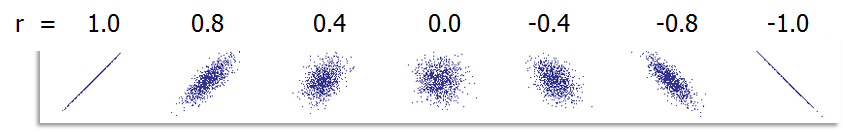## Correlation Coefficient with Minitab

Pearson’s Correlation Coefficient Pearson’s correlation coefficient is also called Pearson’s r or coefficient of correlation and Pearson’s product moment correlation coefficient (r), where r is a statistic measuring the linear relationship between two variables. What is Correlation? Correlation is a statistical technique that describes whether and how strongly two or more variables are related. Correlation [...]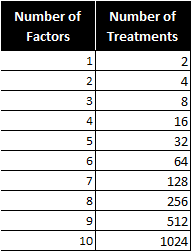## Fractional Factorial Designs with JMP

What Are Fractional Factorial Experiments? In simple terms, a fractional factorial experiment is a subset of a full factorial experiment. Fractional factorials use fewer treatment combinations and runs Fractional factorials are less able to determine effects because of fewer degrees of freedom available to evaluate higher order interactions Fractional factorials can be used to screen [...]## Fractional Factorial Designs with SigmaXL

What Are Fractional Factorial Experiments? In simple terms, a fractional factorial experiment is a subset of a full factorial experiment. Fractional factorials use fewer treatment combinations and runs. Fractional factorials are less able to determine effects because of fewer degrees of freedom available to evaluate higher order interactions. Fractional factorials can be used to screen [...]## Fractional Factorial Designs with Minitab

What Are Fractional Factorial Experiments? In simple terms, a fractional factorial experiment is a subset of a full factorial experiment. Fractional factorials use fewer treatment combinations and runs. Fractional factorials are less able to determine effects because of fewer degrees of freedom available to evaluate higher order interactions. Fractional factorials can be used to screen [...]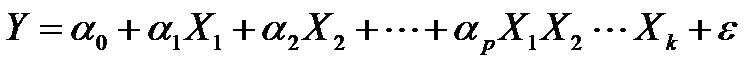## Full Factorial DOE with Minitab

What is a Full Factorial DOE? In a full factorial experiment, all of the possible combinations of factors and levels are created and tested. For example, for two-level design (i.e.each factor has two levels) with k factors, there are 2k possible scenarios or treatments. Two factors, each with two levels, we have 22 = 4 treatments [...]## Full Factorial DOE with SigmaXL

What is a Full Factorial DOE? In a full factorial experiment, all of the possible combinations of factors and levels are created and tested. For example, for two-level design (i.e.each factor has two levels) with k factors, there are 2k possible scenarios or treatments. Two factors, each with two levels, we have 22 = 4 treatments [...]## Full Factorial DOE with JMP

Full Factorial DOE In a full factorial experiment, all of the possible combinations of factors and levels are created and tested. For example, for two-level design (i.e.each factor has two levels) with k factors, there are 2k possible scenarios or treatments. Two factors, each with two levels, we have 22 = 4 treatments Three factors, each [...]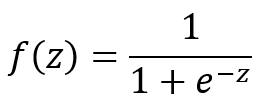## Logistic Regression with JMP

What is Logistic Regression? Logistic regression is a statistical method to predict the probability of an event occurring by fitting the data to a logistic curve using logistic function. The regression analysis used for predicting the outcome of a categorical dependent variable, based on one or more predictor variables. The logistic function used to model [...]## Logistic Regression with Minitab

What is Logistic Regression? Logistic regression is a statistical method to predict the probability of an event occurring by fitting the data to a logistic curve using logistic function. The regression analysis used for predicting the outcome of a categorical dependent variable, based on one or more predictor variables. The logistic function used to model [...]## Logistic Regression with SigmaXL

What is Logistic Regression? Logistic regression is a statistical method to predict the probability of an event occurring by fitting the data to a logistic curve using logistic function. The regression analysis used for predicting the outcome of a categorical dependent variable, based on one or more predictor variables. The logistic function used to model [...]## Stepwise Regression with JMP

What is Stepwise Regression? Stepwise regression is a statistical method to automatically select regression models with the best sets of predictive variables from a large set of potential variables. There are different statistical methods used in stepwise regression to evaluate the potential variables in the model: F-test T-test R-square AIC Three Approaches to Stepwise Regression [...]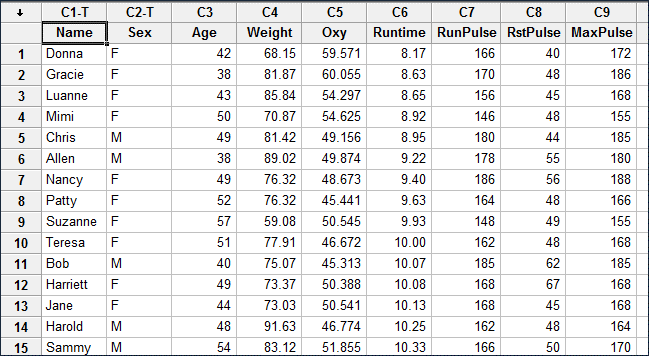## Stepwise Regression with Minitab

What is Stepwise Regression? Stepwise regression is a statistical method to automatically select regression models with the best sets of predictive variables from a large set of potential variables. There are different statistical methods used in stepwise regression to evaluate the potential variables in the model: F-test T-test R-square AIC Three Approaches to Stepwise Regression [...]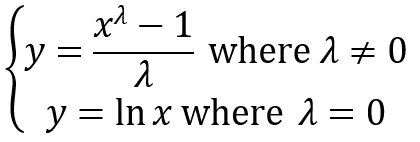## Box Cox Transformation with JMP

What is a Box Cox Transformation? Data transforms are usually applied so that the data appear to more closely meet assumptions of a statistical inference model to be applied or to improve the interpret-ability or appearance of graphs. Power transformation is a class of transformation functions that raise the response to some power. For example, a [...]## Box Cox Transformation with SigmaXL

Box Cox Transformation Data transforms are usually applied so that the data appear to more closely meet assumptions of a statistical inference model to be applied or to improve the interpret-ability or appearance of graphs. Power transformation is a class of transformation functions that raise the response to some power. For example, a square root [...]## Box Cox Transformation with Minitab

What is a Box Cox Transformation? Box Cox Transformation Formula The formula of the Box Cox transformation is: Where: Use Minitab to Perform a Box-Cox Transformation Minitab provides the best Box-Cox transformation with an optimal λ that minimizes the model SSE (sum of squared error). Here is an example of how we transform the non-normally […]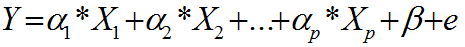## Multiple Linear Regression with Minitab

What is Multiple Linear Regression? Multiple linear regression is a statistical technique to model the relationship between one dependent variable and two or more independent variables by fitting the data set into a linear equation. The difference between simple linear regression and multiple linear regression: Simple linear regression only has one predictor. Multiple linear regression [...]## Multiple Linear Regression with SigmaXL

What is Multiple Linear Regression? Multiple linear regression is a statistical technique to model the relationship between one dependent variable and two or more independent variables by fitting the data set into a linear equation. The difference between simple linear regression and multiple linear regression: Simple linear regression only has one predictor. Multiple linear regression [...]## Multiple Linear Regression with JMP

What is Multiple Linear Regression? Multiple linear regression is a statistical technique to model the relationship between one dependent variable and two or more independent variables by fitting the data set into a linear equation. The difference between simple linear regression and multiple linear regression: Simple linear regression only has one predictor Multiple linear regression [...]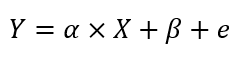## Simple Linear Regression with JMP

What is Simple Linear Regression? Simple linear regression is a statistical technique to fit a straight line through the data points. It models the quantitative relationship between two variables. It is simple because only one predictor variable is involved. It describes how one variable changes according to the change of another variable. Both variables need [...]